Algebra Commons™

1,047 Full-Text Articles 1,224 Authors 224,226 Downloads118 Institutions

All Articles in Algebra

1,047 full-text articles. Page 5 of 39.

Determinants Of Interval Matrices, 2018Charles University, Prague, Czech Republic

Determinants Of Interval Matrices, Jaroslav Horáček, Milan Hladík, Josef Matějka

Electronic Journal of Linear Algebra

In this paper we shed more light on determinants of real interval matrices. Computing the exact bounds on a determinant of an interval matrix is an NP-hard problem. Therefore, attention is first paid to approximations. NP-hardness of both relative and absolute approximation is proved. Next, methods computing verified enclosures of interval determinants and their possible combination with preconditioning are discussed. A new method based on Cramer's rule was designed. It returns similar results to the state-of-the-art method, however, it is less consuming regarding computational time. Other methods transferable from real matrices (e.g., the Gerschgorin circles, Hadamard's inequality ...

2018Huaibei Normal University

Inequalities Between $\Mid A\Mid + \Mid B\Mid$ And $\Mid A^{*} \Mid + \Mid B^{*} \Mid$, Yun Zhang

Electronic Journal of Linear Algebra

Let $A$ and $B$ be complex square matrices. Some inequalities between $\mid A \mid + \mid B \mid$ and $\mid A^{*} \mid + \mid B^{*} \mid$ are established. Applications of these inequalities are also given. For example, in the Frobenius norm, $$\parallel\, A+B \,\parallel_{F} \leq \sqrt{2} \parallel \mid A\mid + \mid B\mid \, \parallel_{F}.$$

2018Georgia State University

33 - On The Existence Of An Arbitrarily Large Number Of Generators For The Presentation Ideal Of A Semigroup Ring., Arun Suresh

Georgia Undergraduate Research Conference (GURC)

Consider K to be an arbitrary field, and P(n1,…, nm) be the ideal of polynomials given by

P(n1,…, nm) = {f(x1, … , xm) : f(x1,…,xm) ∈ K[x1,…,xm], f(tn1, … ,tnm) = 0, where t is transcendental over K}.

In 1970, J. Herzog showed that the least upper bound on the number of generators of K, for m = 3, is 3. It can be lowered to two, if n1, n2, n3 satisfy a few symmetry conditions. Following that, Bresinsky in 1975, showed ...

About A Non-Standard Interpolation Problem, 2018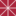Chapman University

About A Non-Standard Interpolation Problem, Daniel Alpay, Alain Yger

Mathematics, Physics, and Computer Science Faculty Articles and Research

Using algebraic methods, and motivated by the one variable case, we study a multipoint interpolation problem in the setting of several complex variables. The duality realized by the residue generator associated with an underlying Gorenstein algebra, using the Lagrange interpolation polynomial, plays a key role in the arguments.

On Projection Of A Positive Definite Matrix On A Cone Of Nonnegative Definite Toeplitz Matrices, 2018Poznań University Of Technology

On Projection Of A Positive Definite Matrix On A Cone Of Nonnegative Definite Toeplitz Matrices, Katarzyna Filipiak, Augustyn Markiewicz, Adam Mieldzioc, Aneta Sawikowska

Electronic Journal of Linear Algebra

We consider approximation of a given positive definite matrix by nonnegative definite banded Toeplitz matrices. We show that the projection on linear space of Toeplitz matrices does not always preserve nonnegative definiteness. Therefore we characterize a convex cone of nonnegative definite banded Toeplitz matrices which depends on the matrix dimensions, and we show that the condition of positive definiteness given by Parter [{\em Numer. Math. 4}, 293--295, 1962] characterizes the asymptotic cone. In this paper we give methodology and numerical algorithm of the projection basing on the properties of a cone of nonnegative definite Toeplitz matrices. This problem can be ...

Explicit Block-Structures For Block-Symmetric Fiedler-Like Pencils, 2018University of California, Santa Barbara

Explicit Block-Structures For Block-Symmetric Fiedler-Like Pencils, M. I. Bueno, Madeline Martin, Javier Perez, Alexander Song, Irina Viviano

Electronic Journal of Linear Algebra

In the last decade, there has been a continued effort to produce families of strong linearizations of a matrix polynomial $P(\lambda)$, regular and singular, with good properties, such as, being companion forms, allowing the recovery of eigenvectors of a regular $P(\lambda)$ in an easy way, allowing the computation of the minimal indices of a singular $P(\lambda)$ in an easy way, etc. As a consequence of this research, families such as the family of Fiedler pencils, the family of generalized Fiedler pencils (GFP), the family of Fiedler pencils with repetition, and the family of generalized Fiedler pencils with ...

On The Largest Distance (Signless Laplacian) Eigenvalue Of Non-Transmission-Regular Graphs, 2018East China Normal University

On The Largest Distance (Signless Laplacian) Eigenvalue Of Non-Transmission-Regular Graphs, Shuting Liu, Jinlong Shu, Jie Xue

Let $G=(V(G),E(G))$ be a $k$-connected graph with $n$ vertices and $m$ edges. Let $D(G)$ be the distance matrix of $G$. Suppose $\lambda_1(D)\geq \cdots \geq \lambda_n(D)$ are the $D$-eigenvalues of $G$. The transmission of $v_i \in V(G)$, denoted by $Tr_G(v_i)$ is defined to be the sum of distances from $v_i$ to all other vertices of $G$, i.e., the row sum $D_{i}(G)$ of $D(G)$ indexed by vertex $v_i$ and suppose that $D_1(G)\geq \cdots \geq D_n(G)$. The $Wiener~ index$ of $G$ denoted by $W ... Bounded Linear Operators That Preserve The Weak Supermajorization On$\Ell^1(I)^+$, 2018Faculty of Mechanical Engineering, Department of Mathematics,University of Niš, Serbia Bounded Linear Operators That Preserve The Weak Supermajorization On$\Ell^1(I)^+$, Martin Z. Ljubenović, Dragan S. Djordjevic Electronic Journal of Linear Algebra Linear preservers of weak supermajorization which is defined on positive functions contained in the discrete Lebesgue space$\ell^1(I)\$ are characterized. Two different classes of operators that preserve the weak supermajorization are formed. It is shown that every linear preserver may be decomposed as sum of two operators from the above classes, and conversely, the sum of two operators which satisfy an additional condition is a linear preserver. Necessary and sufficient conditions under which a bounded linear operator is a linear preserver of the weak supermajorization are given. It is concluded that positive linear preservers of the weak supermajorization ...

Math Active Learning Lab: Math 93 Notebook, 2018University of North Dakota

Math Active Learning Lab: Math 93 Notebook, Michele Iiams, Gwennie Byron

Open Educational Resources

This course notebook has been designed for students of Math 93 (Algebra Prep III) at the University of North Dakota. It has been designed to help you get the most out of the ALEKS resources and your time.

• Topics in the Notebook are organized by weekly learning module.
• Space for notes from ALEKS learning pages, e-book and videos directs you to essential concepts.
• Examples and “You Try It” problems have been carefully chosen to help you focus on these essential concepts.
• Completed Notebook is an invaluable tool when studying for exams.

Otto Holder's Formal Christening Of The Quotient Group Concept, 2018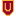Colorado State University-Pueblo

Otto Holder's Formal Christening Of The Quotient Group Concept, Janet Heine Barnett

Abstract Algebra

No abstract provided.

College Algebra (Abac), 2018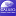Abraham Baldwin Agricultural College

College Algebra (Abac), April Abbott, Gary Dicks, Jan Gregus, Buddhi Pantha, Melanie Partlow, Lori Pearman, Amanda Urquhart, Eunkyung You

Mathematics Grants Collections

This Grants Collection for College Algebra was created under a Round Ten ALG Textbook Transformation Grant.

Affordable Learning Georgia Grants Collections are intended to provide faculty with the frameworks to quickly implement or revise the same materials as a Textbook Transformation Grants team, along with the aims and lessons learned from project teams during the implementation process.

Documents are in .pdf format, with a separate .docx (Word) version available for download. Each collection contains the following materials:

• Initial Proposal
• Final Report

College Algebra (Asu), 2018Albany State University

College Algebra (Asu), Zephyrinus Okonkwo, Anilkumar Deverapu, Anthony Smith, Vijay Kunwar, Laxmi Paudel

Mathematics Grants Collections

This Grants Collection for College Algebra was created under a Round Ten ALG Textbook Transformation Grant.

Affordable Learning Georgia Grants Collections are intended to provide faculty with the frameworks to quickly implement or revise the same materials as a Textbook Transformation Grants team, along with the aims and lessons learned from project teams during the implementation process.

Documents are in .pdf format, with a separate .docx (Word) version available for download. Each collection contains the following materials:

• Initial Proposal
• Final Report

Deblurring Images, 2018Western Washington University

Deblurring Images, Jamie Mcmullen

WWU Honors Program Senior Projects

Let the matrix B be a blurred version of a sharp image represented by the matrix X. Given B, we would like to recover X.

To accomplish this, we construct linear models of the blurring process that produced B from X. The idea is that we could then reverse the blurring to reproduce the original image.

For example, if the blurred image satisfies

B = CXRT

for some invertible matrices C and R, then we could recover X as

X = C-1B(RT)-1.

However, the blurring model usually fails to account for all the blurring that actually ...

2018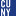The Graduate Center, City University of New York

Galois Groups Of Differential Equations And Representing Algebraic Sets, Eli Amzallag

All Dissertations, Theses, and Capstone Projects

The algebraic framework for capturing properties of solution sets of differential equations was formally introduced by Ritt and Kolchin. As a parallel to the classical Galois groups of polynomial equations, they devised the notion of a differential Galois group for a linear differential equation. Just as solvability of a polynomial equation by radicals is linked to the equation’s Galois group, so too is the ability to express the solution to a linear differential equation in "closed form" linked to the equation’s differential Galois group. It is thus useful even outside of mathematics to be able to compute and ...

Math Active Learning Lab: Math 92 Notebook, 2018University of North Dakota

Math Active Learning Lab: Math 92 Notebook, Michele Iiams, Gwennie Byron

Open Educational Resources

This course notebook has been designed for students of Math 92 (Algebra Prep II) at the University of North Dakota. It has been designed to help you get the most out of the ALEKS resources and your time.

• Topics in the Notebook are organized by weekly learning module.
• Space for notes from ALEKS learning pages, e-book and videos directs you to essential concepts.
• Examples and “You Try It” problems have been carefully chosen to help you focus on these essential concepts.
• Completed Notebook is an invaluable tool when studying for exams.

2018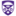The University of Western Ontario

High Performance Sparse Multivariate Polynomials: Fundamental Data Structures And Algorithms, Alex Brandt

Electronic Thesis and Dissertation Repository

Polynomials may be represented sparsely in an effort to conserve memory usage and provide a succinct and natural representation. Moreover, polynomials which are themselves sparse – have very few non-zero terms – will have wasted memory and computation time if represented, and operated on, densely. This waste is exacerbated as the number of variables increases. We provide practical implementations of sparse multivariate data structures focused on data locality and cache complexity. We look to develop high-performance algorithms and implementations of fundamental polynomial operations, using these sparse data structures, such as arithmetic (addition, subtraction, multiplication, and division) and interpolation. We revisit a sparse ...

2018University of South Carolina Aiken

The Largest Eigenvalue And Some Hamiltonian Properties Of Graphs, Rao Li

Electronic Journal of Linear Algebra

In this note, sufficient conditions, based on the largest eigenvalue, are presented for some Hamiltonian properties of graphs.

Proof Of A Conjecture Of Graham And Lovasz Concerning Unimodality Of Coefficients Of The Distance Characteristic Polynomial Of A Tree, 2018Iowa State University

Proof Of A Conjecture Of Graham And Lovasz Concerning Unimodality Of Coefficients Of The Distance Characteristic Polynomial Of A Tree, Ghodratollah Aalipour, Aida Abiad, Zhanar Berikkyzy, Leslie Hogben, Franklin H.J. Kenter, Jephian C.-H. Lin, Michael Tait

Electronic Journal of Linear Algebra

The conjecture of Graham and Lov ́asz that the (normalized) coefficients of the distance characteristic polynomial of a tree are unimodal is proved; it is also shown that the (normalized) coefficients are log-concave. Upper and lower bounds on the location of the peak are established.

Extremal Octagonal Chains With Respect To The Spectral Radius, 2018Anhui University of Science and Technology

Extremal Octagonal Chains With Respect To The Spectral Radius, Xianya Geng, Shuchao Li, Wei Wei

Electronic Journal of Linear Algebra

Octagonal systems are tree-like graphs comprised of octagons that represent a class of polycyclic conjugated hydrocarbons. In this paper, a roll-attaching operation for the calculation of the characteristic polynomials of octagonal chain graphs is proposed. Based on these characteristic polynomials, the extremal octagonal chains with n octagons having the maximum and minimum spectral radii are identified.

The Effects Of Motivation, Technology And Satisfaction On Student Achievement In Face-To-Face And Online College Algebra Classes, 2018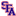South Texas College

The Effects Of Motivation, Technology And Satisfaction On Student Achievement In Face-To-Face And Online College Algebra Classes, Hanan Jamal Amro, Marie-Anne Mundy, Lori Kupczynski

TxDLA Journal of Distance Learning

Demand for online learning has increased in recent years due to the convenience of class delivery. However, some students appear to have difficulties with online education resulting in lack of completion. The study utilized a quantitative approach with archival data and survey design. The factors of demographics, motivation, technology, and satisfaction were compared for face-to-face and online students. MANCOVA tests were performed to analyze the data while controlling age and gender to uncover significant differences between the two groups. The sample and population for this study were predominantly Hispanic students.

Motivation and Technology were non-significant, but satisfaction was proven to ...